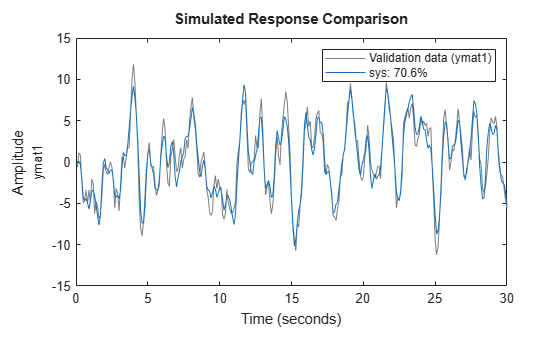# Convert SISO Matrix Data to Timetable

Load the data, which consists of the input vector `umat1`, the output vector `ymat1`, and the sample time `Ts`.

`load sdata1 umat1 ymat1 Ts`

Combine the data into the single timetable `tt`. View the first two rows of `tt`.

```tt = timetable(umat1,ymat1,'rowtimes',seconds(Ts*(1:size(umat1,1)))); head(tt,2)```
``` Time umat1 ymat1 _______ _____ ________ 0.1 sec 1 -0.58724 0.2 sec -1 1.1082 ```

Use `tt` to estimate a continuous-time state-space model.

`sys = ssest(tt,2);`

Compare the model output with the estimation data.

`compare(tt,sys)`